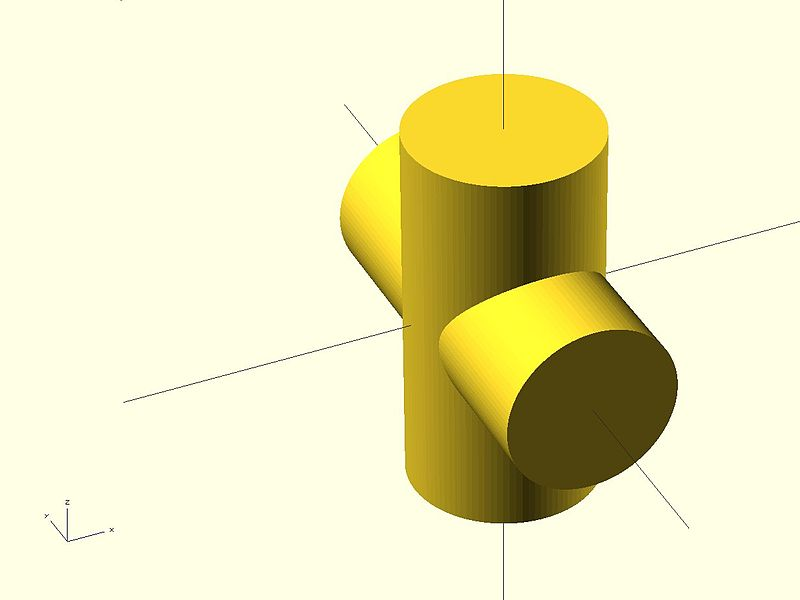2015/02/09 16:20

### Union：合并

Usage example:

union() {
cylinder (h = 4, r=1, center = true, $fn=100); rotate ([90,0,0]) cylinder (h = 4, r=0.9, center = true,$fn=100);
}

注意: union是隐含的，当未使用时。但当两个对象相交时，就成为必然的操作。### difference：切割

从主对象中把所有字对象挖出，如下所示：

Usage example:

difference() {
cylinder (h = 4, r=1, center = true, $fn=100); rotate ([90,0,0]) cylinder (h = 4, r=0.9, center = true,$fn=100);
}

### intersection：求交

创建所有子对象的交集，保留重叠的部分。如下所示：

Usage example:

intersection() {
cylinder (h = 4, r=1, center = true, $fn=100); rotate ([90,0,0]) cylinder (h = 4, r=0.9, center = true,$fn=100);
}

### render：渲染

Usage example:
render(convexity = 1) { ... }

 convexity Integer. The convexity parameter specifies the maximum number of front sides (back sides) a ray intersecting the object might penetrate. This parameter is only needed for correctly displaying the object in OpenCSG preview mode and has no effect on the polyhedron rendering.

This image shows a 2D shape with a convexity of 4, as the ray indicated in red crosses the 2D shape a maximum of 4 times. The convexity of a 3D shape would be determined in a similar way. Setting it to 10 should work fine for most cases.### 作者的其它热门文章

0
2 收藏

0 评论
2 收藏
0